# 一种基于入侵杂草算法改进的差分进化算法A Modified Differential Evolution Algorithm Based on Invasive Weed Optimization

• 全文下载: PDF(579KB)    PP.266-274   DOI: 10.12677/CSA.2019.92031
• 下载量: 151  浏览量: 260

DE/rand/1/bin model of DE Algorithm has good global performance, but its convergence speed is slow. A modified differential evolution optimization algorithm named IWOMDE was presented in this paper based on Invasive Weed Optimization algorithm. The IWOMDE algorithm incorporated IWO’s design philosophy into Differential Evolution (DE) algorithm. The IWOMDE algorithm divided the evolution population into elite individuals and poor individuals based on the average fitness of population. The elite individuals can be evolutionary many times, but the poor individuals are less evolved or “stagnate” in order to maintain the diversity of population. The simulation results showed the hybrid optimization algorithm has the advantage of searching effectively and being fairly robust to initial conditions.

1. 引言

2. IWOMDE算法

2.1. 差分进化算法

DE算法的进化个体采用实向量进行编码，采用均匀分布的随机数产生初始个体。令 ${x}_{i}\left(g\right)$ 代表第g代的第i个个体， ${x}_{i}^{L}\le {x}_{i}\left(g\right)\le {x}_{i}^{U}$ ，则

${x}_{i}\left(g\right)=\left({x}_{i1}\left(g\right),{x}_{i2}\left(g\right),\cdots ,{x}_{in}\left(g\right)\right),\text{\hspace{0.17em}}\text{\hspace{0.17em}}i=1,2,\cdots ,\text{NP};\text{\hspace{0.17em}}g=1,2,\cdots ,{T}_{\mathrm{max}}$ (1)

DE算法就是由这NP个个体构成的种群在搜索空间不断进化来进行寻优的。DE算法候选解产生模式有很多种策略  ，其中DE/rand/1/bin策略应用较为广泛，且具有全局搜索能力较好，收敛速度较慢的特性，因此，本文选择该进化模式为研究对象。以求解最小值问题为例说明差分进化算法的进化过程如下。

1) 初始化种群

${x}_{ij}\left(0\right)={x}_{ij}^{L}+rand\left(0,1\right)\left({x}_{ij}^{U}-{x}_{ij}^{L}\right)$ (2)

2) 变异算子

${h}_{ij}\left(g\right)={x}_{p1j}+F\left({x}_{p2j}-{x}_{p3j}\right)$ (3)

3) 交叉算子

${v}_{ij}\left(g+1\right)=\left\{\begin{array}{l}{h}_{i}{}_{j}\left(g\right),rand\left(0,1\right)\le \text{CR}\text{ }或\text{ }j={j}_{rand}\\ {x}_{ij}\left(g\right),rand\left(0,1\right)>\text{CR}\text{ }或\text{ }j\ne {j}_{rand}\end{array}$ (4)

4) 选择算子

DE算法的选择策略采用“贪婪”策略，由评价函数对向量 ${v}_{i}\left(g+1\right)$ 和向量 ${x}_{i}\left(g\right)$ 比较，保留较优个体，即

${x}_{i}\left(g+1\right)=\left\{\begin{array}{l}{v}_{i}\left(g+1\right),f\left({v}_{i}\left(g+1\right)\right) (5)

2.2. IWOMDE算法

DE算法的变异策略有多种，其中DE/rand/1/bin变异策略具有全局搜索能力强、收敛速度慢的特性。由式(3)可知，DE/rand/1/bin变异过程中，其候选解的产生是采用的是种群中随机选择的3个不同个体，通过线性运算产生的。该变异过程并没有考虑所选择个体的适应度信息，随机性强，有利于保持种群的多样性，但同时也使算法的收敛速度较慢。

DE算法的随机搜索过程，使得算法的局部搜索能力较差，收敛速度慢，这主要是由于进化过程中，尤其是在候选解的产生过程中未考虑个体的适应度引起的，因此，借鉴IWO算法的进化思想，将适应度的信息融入候选解的生成过程，使较优的个体能够产生较多的候选解，较差的个体产生较少的候选解或者保持停滞状态。这样不但能够使最优个体引导群体快速收敛，而且由于有个体随机停滞现象，能够很好地保持群体的多样性。

1) 优秀个体的选取

${f}_{avg}=\frac{1}{\text{NP}}\underset{i=1}{\overset{\text{NP}}{\sum }}{f}_{i}$ (6)

2) 修正的变异算子

Step1：变异算子

if $\left(rand\left(0,1\right)<{P}_{1}\right)$

${h}_{ij}\left(g\right)=\left[1+\sigma \left(0,1\right)\right]{x}_{ij}+F\left({x}_{gbestj}-{x}_{ij}\right)$ (7)

else

${h}_{ij}\left(g\right)={x}_{p1j}+F\left({x}_{p2j}-{x}_{p3j}\right)$

Step2：执行式(4)的交叉算子

Step3：执行式(5)的选择算子

Step4：if $\left[f\left({x}_{i}\left(g+1\right)\right) ，转Step1继续进化。

if $\left(rand\left(0,1\right)<1-{P}_{1}\right)$

${h}_{ij}\left(g\right)={x}_{p1j}+F\left({x}_{p2j}-{x}_{p3j}\right)$

else

Step1：设置种群规模NP、交叉概率CR、缩放因子F，计算精度 $\epsilon$ 及优秀个体进化概率 ${P}_{1}$ ，在参数空间随机初始化每一个个体，设置最大进化代数为T，令 $t=1$

Step2：计算当前种群的最优适应度bestfitness及最优个体 ${x}_{gbest}$

Step3：若最优适应度bestfitness达到精度要求或者迭代次数达到最大，则输出当前最优适应度值，退出；否则，转Step4。

Step4：依据式(6)计算当前群体的平均适应度；

Step5：对群体中的所有个体执行

${f}_{i}<{f}_{avg}$ 按优秀个体进行进化；否则，按较差个体进化。

Step6 $t=t+1$ ，转Step2。

3. 仿真结果及分析

3.1. 仿真测试设置

${f}_{1}=0.5-\frac{\left({\mathrm{sin}}^{2}\sqrt{{x}_{1}^{2}+{x}_{2}^{2}}-0.5\right)}{{\left(1+0.001\left({x}_{1}^{2}+{x}_{2}^{2}\right)\right)}^{2}}\text{}-100\le {x}_{1},{x}_{2}\le 100,$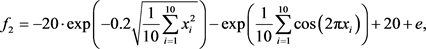$-32.768\le {x}_{i}\le 32.768$

${f}_{3}=\underset{i=1}{\overset{29}{\sum }}\left(100{\left({x}_{i}^{2}-{x}_{i+1}^{2}\right)}^{2}+{\left({x}_{i}-1\right)}^{2}\right)\text{}-2.048\le {x}_{i}\le 2.048,$

${f}_{4}=\underset{i=1}{\overset{30}{\sum }}\left({x}_{i}-10\mathrm{cos}\left(2\text{π}{x}_{i}\right)+10\right)\text{}-5.12\le {x}_{i}\le 5.12,$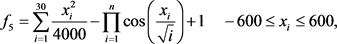Table 1. Experimental parameter setting of algorithm

3.2. 测试结果及分析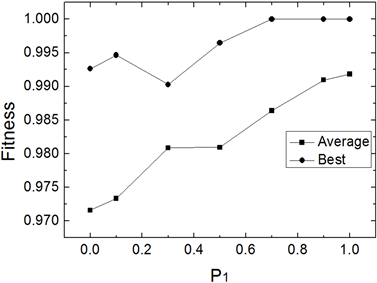Figure 1. Comparison of mean fitness and optimal fitness of 30 runs at different P1 of f1(x)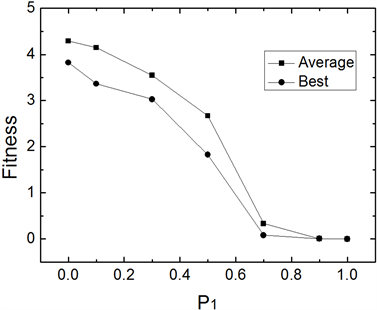Figure 2. Comparison of mean fitness and optimal fitness of 30 runs at different P1 of f2(x)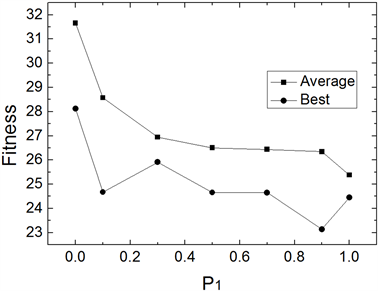Figure 3. Comparison of mean fitness and optimal fitness of 30 runs at different P1 of f3(x)Table 2. Comparison of the optimization results of functions by IWOMDE and SMDETable 3. Comparison of the optimization results of functions by IWOMDE and ADETable 4. Comparison of the optimization results of functions by IWOMDE and ONDE

4. 结语

DE算法的DE/rand/1/bin进化模式具有全局搜索能力的特性，被广泛应用于实际问题求解中，但其收敛速度较慢，局部搜索能较差。为使DE算法能够实现快速收敛，且具有很强的搜索精度，本文提出了一种简单易实现的IWOMDE算法，该算法受入侵杂草算法(IWO)启发，将进化群体依据群体适应度均值进行划分为优秀个体与较差个体，对两种不同个体采用不同的变异算子。多个Benchmarks函数的优化结果表明，IWOMDE具有收敛速度快，对于多峰函数有较强的优化效率，但对于单峰病态函数优化效率不高的特点，可广泛应用于各种实际工程优化问题中。

  Storn, R. and Price, K. (1997) Differential Evolution—A Simple and Efficient Adaptive Scheme for Global Optimization over Continuous Spaces. Journal of Global Optimization, 11, 341-359. https://doi.org/10.1023/A:1008202821328  刘波, 王凌, 金以慧. 差分进化算法研究进展[J]. 控制与决策, 2007, 22(7): 721-729.  Vesterstrom, J. and Thomsen, R. (2004) A Comparative Study of Differential Evolution, Particle Swarm Optimization, and Evolutionary Algorithms on Numerical Benchmark Problems. Proceedings of the 2004 Congress on Evolutionary Computation, Portland, 19-23 June 2004, 1980-1987. https://doi.org/10.1109/CEC.2004.1331139  卢青波, 张学良, 温淑花, 等. 差异演化算法改进与应用[J]. 农业机械学报, 2010, 41(2): 193-197  温淑花, 张学良, 卢青波, 等. 差异演化算法及其在机械优化设计中的应用[J]. 农业机械学报, 2008, 39(8): 135-139.  卢青波, 张学良, 温淑花, 等. 基于差异演化算法的动压滑动轴承多目标优化[J]. 农业机械学报, 2013, 44(3): 230-236, 245.  陈华, 范宜仁, 邓少贵. 基于Logistic模型的自适应差分进化算法[J]. 控制与决策, 2011, 26(7): 1105-1108.  Fu, H.J., Ouyang, D.T. and Xu, J.M. (2011) A Self-Adaptive Differential Evolution Algorithm for Binary CSPS. Computers & Mathematics with Applications, 62, 2712-2718. https://doi.org/10.1016/j.camwa.2011.06.053  刘洁, 吴亮红, 刘建勋. 基于单纯形算子的混合差分进化算法[J]. 计算机工程，2009, 35(13): 179-182.  阳春华, 钱晓山, 桂卫华. 一种混沌差分进化和粒子群优化混合算法[J]. 计算机应用研究, 2011, 28(2): 439-441.  Mehrabian, A.R. and Lucas, C. (2006) A Novel Numerical Optimization Algorithm Inspired from Weed Optimization. Ecological Informaticas, 1, 355-366. https://doi.org/10.1016/j.ecoinf.2006.07.003  拓守恒, 汪文勇. 求解高维多模优化问题的正交小生境自适应差分演化算法[J]. 计算机应用, 2011, 31(4): 1094-1098.